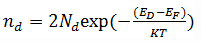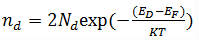Courses

# Test: Donor And Acceptor Impurities

## 10 Questions MCQ Test Electronic Devices | Test: Donor And Acceptor Impurities

Description
This mock test of Test: Donor And Acceptor Impurities for Electrical Engineering (EE) helps you for every Electrical Engineering (EE) entrance exam. This contains 10 Multiple Choice Questions for Electrical Engineering (EE) Test: Donor And Acceptor Impurities (mcq) to study with solutions a complete question bank. The solved questions answers in this Test: Donor And Acceptor Impurities quiz give you a good mix of easy questions and tough questions. Electrical Engineering (EE) students definitely take this Test: Donor And Acceptor Impurities exercise for a better result in the exam. You can find other Test: Donor And Acceptor Impurities extra questions, long questions & short questions for Electrical Engineering (EE) on EduRev as well by searching above.
QUESTION: 1

### At what temperature the donor states are completely ionized?

Solution:

At room temperature, the donors have donated their electrons to the conduction band.

QUESTION: 2

### The opposite of ionization takes place at which temperature?

Solution:

AT 0 K, all the donors and acceptors are in their lowest energy levels.

QUESTION: 3

### What do you mean by the tem ‘FREEZE-OUT’?

Solution:

Freeze out means none of the electrons are transmitted to the conduction band.

QUESTION: 4

Which of the following expressions represent the correct formula for the density of electrons occupying the donor level?

Solution:

The density of the electrons is equal to the electrons present in the substrate minus the number of donors present.

QUESTION: 5

Which of the following band is just above the intrinsic Fermi level for n-type semiconductor?

Solution:

For n-type semiconductors, the donor band is just above the intrinsic Fermi level.

QUESTION: 6

At absolute zero temperature, which level is above the Fermi energy level in the case of donors?

Solution:

At T=0 K, the tem exp(-∞)=0 in the expression ofThus, EF>ED
So, only conduction band lies above the Fermi energy level.

QUESTION: 7

At T=0 K, the location of Fermi level with respect to the Ec and Ed for the n type material is?

Solution:

At T=0 K, the Fermi level of n band lies between the midway of Ec and Ed as intrinsic Fermi level always lies between the Ec and Ev.

QUESTION: 8

At absolute zero temperature, which level is below the Fermi energy level in the case of acceptors?

Solution:

At T=0 K, the tem exp(-∞)=0 in the expression ofSo, only valence band lies below the Fermi energy level of the acceptors.

QUESTION: 9For the above n-type semiconductor, what is B knows as?

Solution:

For n-type semiconductors, the donor energy level is always greater than the Fermi level energy.

QUESTION: 10For the above given figure, identify the correct option for satisfying the above semiconductor figure?

Solution:

The given figure has B band below the intrinsic Fermi level, so that would be acceptor energy band and will be a p-type semiconductor.